Publicité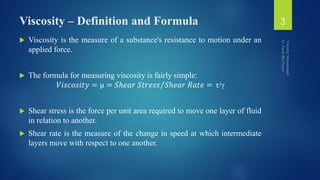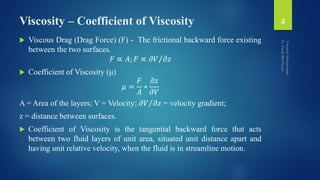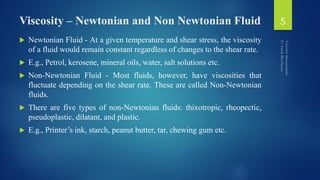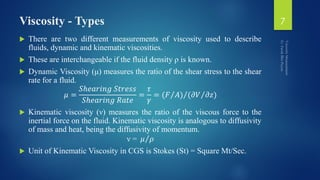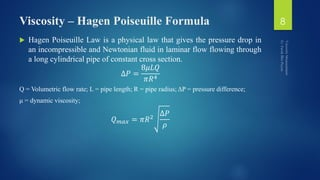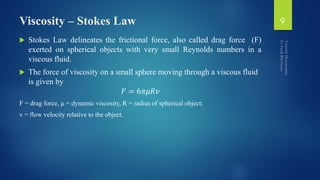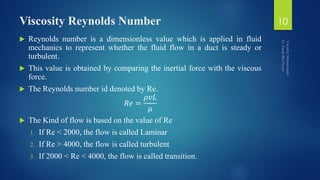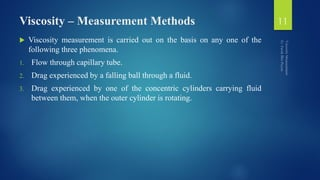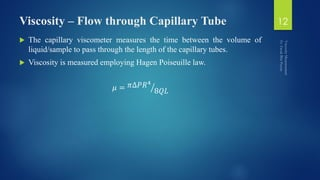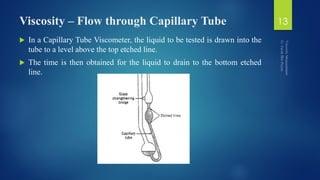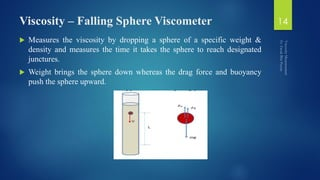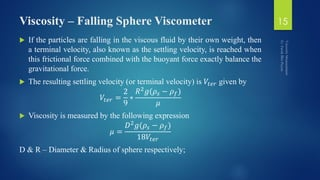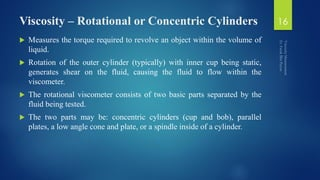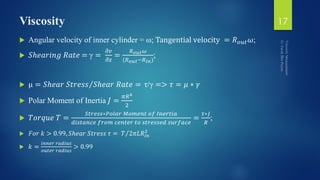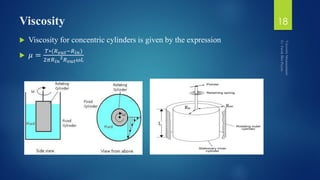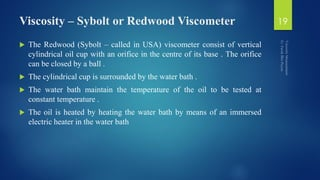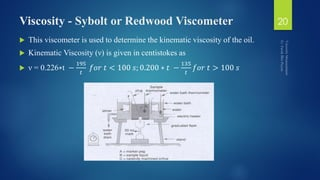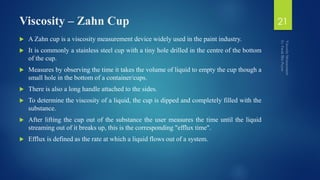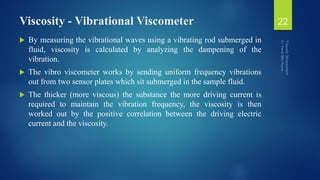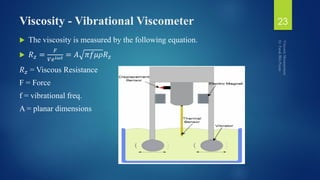1 sur 25
Publicité

### Viscosity Measurement

1. Viscosity Measurement ER. FARUK BIN POYEN FARUK.POYEN@GMAIL.COM
2. Content:  Definition  Coefficient  Units  Measuring Devices 2
3. Viscosity – Definition and Formula  Viscosity is the measure of a substance's resistance to motion under an applied force.  The formula for measuring viscosity is fairly simple: 𝑉𝑖𝑠𝑐𝑜𝑠𝑖𝑡𝑦 = μ = 𝑆ℎ𝑒𝑎𝑟 𝑆𝑡𝑟𝑒𝑠𝑠 𝑆ℎ𝑒𝑎𝑟 𝑅𝑎𝑡𝑒 = τ/γ  Shear stress is the force per unit area required to move one layer of fluid in relation to another.  Shear rate is the measure of the change in speed at which intermediate layers move with respect to one another. 3
4. Viscosity – Coefficient of Viscosity  Viscous Drag (Drag Force) (F) - The frictional backward force existing between the two surfaces. 𝐹 ∝ 𝐴; 𝐹 ∝ 𝜕𝑉 𝜕𝑧  Coefficient of Viscosity (μ) 𝜇 = 𝐹 𝐴 ∗ 𝜕𝑧 𝜕𝑉 A = Area of the layers; V = Velocity; 𝜕𝑉 𝜕𝑧 = velocity gradient; z = distance between surfaces.  Coefficient of Viscosity is the tangential backward force that acts between two fluid layers of unit area, situated unit distance apart and having unit relative velocity, when the fluid is in streamline motion. 4
5. Viscosity – Newtonian and Non Newtonian Fluid  Newtonian Fluid - At a given temperature and shear stress, the viscosity of a fluid would remain constant regardless of changes to the shear rate.  E.g., Petrol, kerosene, mineral oils, water, salt solutions etc.  Non-Newtonian Fluid - Most fluids, however, have viscosities that fluctuate depending on the shear rate. These are called Non-Newtonian fluids.  There are five types of non-Newtonian fluids: thixotropic, rheopectic, pseudoplastic, dilatant, and plastic.  E.g., Printer’s ink, starch, peanut butter, tar, chewing gum etc. 5
6. Viscosity – Units of Viscosity  CGS Unit – Poise (P).  Centipoise (cP) = 0.01 Poise.  SI Unit of μ – (kg/mt-s) = 10 Poise.  SI Unit of Viscosity – (Newton*Sec/square mt.) = Poiseuille (PI).  Other Unit – Pascal Second (Pa.s).  1 PI = 10 Poise = 1000 cP  1 cP = 1 mPa.s 6
7. Viscosity - Types  There are two different measurements of viscosity used to describe fluids, dynamic and kinematic viscosities.  These are interchangeable if the fluid density ρ is known.  Dynamic Viscosity (μ) measures the ratio of the shear stress to the shear rate for a fluid. 𝜇 = 𝑆ℎ𝑒𝑎𝑟𝑖𝑛𝑔 𝑆𝑡𝑟𝑒𝑠𝑠 𝑆ℎ𝑒𝑎𝑟𝑖𝑛𝑔 𝑅𝑎𝑡𝑒 = 𝜏 𝛾 = (𝐹 𝐴) ( 𝜕𝑉 𝜕𝑧)  Kinematic viscosity (ν) measures the ratio of the viscous force to the inertial force on the fluid. Kinematic viscosity is analogous to diffusivity of mass and heat, being the diffusivity of momentum. ν = 𝜇 𝜌  Unit of Kinematic Viscosity in CGS is Stokes (St) = Square Mt/Sec. 7
8. Viscosity – Hagen Poiseuille Formula  Hagen Poiseuille Law is a physical law that gives the pressure drop in an incompressible and Newtonian fluid in laminar flow flowing through a long cylindrical pipe of constant cross section. ∆𝑃 = 8𝜇𝐿𝑄 𝜋𝑅4 Q = Volumetric flow rate; L = pipe length; R = pipe radius; ΔP = pressure difference; μ = dynamic viscosity; 𝑄 𝑚𝑎𝑥 = 𝜋𝑅2 ∆𝑃 𝜌 8
9. Viscosity – Stokes Law  Stokes Law delineates the frictional force, also called drag force (F) exerted on spherical objects with very small Reynolds numbers in a viscous fluid.  The force of viscosity on a small sphere moving through a viscous fluid is given by 𝐹 = 6𝜋𝜇𝑅𝑣 F = drag force, μ = dynamic viscosity, R = radius of spherical object; v = flow velocity relative to the object. 9
10. Viscosity Reynolds Number  Reynolds number is a dimensionless value which is applied in fluid mechanics to represent whether the fluid flow in a duct is steady or turbulent.  This value is obtained by comparing the inertial force with the viscous force.  The Reynolds number id denoted by Re. 𝑅𝑒 = 𝜌𝑣𝐿 𝜇  The Kind of flow is based on the value of Re 1. If Re < 2000, the flow is called Laminar 2. If Re > 4000, the flow is called turbulent 3. If 2000 < Re < 4000, the flow is called transition. 10
11. Viscosity – Measurement Methods  Viscosity measurement is carried out on the basis on any one of the following three phenomena. 1. Flow through capillary tube. 2. Drag experienced by a falling ball through a fluid. 3. Drag experienced by one of the concentric cylinders carrying fluid between them, when the outer cylinder is rotating. 11
12. Viscosity – Flow through Capillary Tube  The capillary viscometer measures the time between the volume of liquid/sample to pass through the length of the capillary tubes.  Viscosity is measured employing Hagen Poiseuille law. 𝜇 = 𝜋∆𝑃𝑅4 8𝑄𝐿 12
13. Viscosity – Flow through Capillary Tube  In a Capillary Tube Viscometer, the liquid to be tested is drawn into the tube to a level above the top etched line.  The time is then obtained for the liquid to drain to the bottom etched line. 13
14. Viscosity – Falling Sphere Viscometer  Measures the viscosity by dropping a sphere of a specific weight & density and measures the time it takes the sphere to reach designated junctures.  Weight brings the sphere down whereas the drag force and buoyancy push the sphere upward. 14
15. Viscosity – Falling Sphere Viscometer  If the particles are falling in the viscous fluid by their own weight, then a terminal velocity, also known as the settling velocity, is reached when this frictional force combined with the buoyant force exactly balance the gravitational force.  The resulting settling velocity (or terminal velocity) is 𝑉𝑡𝑒𝑟 given by 𝑉𝑡𝑒𝑟 = 2 9 ∗ 𝑅2 𝑔(𝜌𝑠 − 𝜌 𝑓) 𝜇  Viscosity is measured by the following expression 𝜇 = 𝐷2 𝑔(𝜌𝑠 − 𝜌 𝑓) 18𝑉𝑡𝑒𝑟 D & R – Diameter & Radius of sphere respectively; 15
16. Viscosity – Rotational or Concentric Cylinders  Measures the torque required to revolve an object within the volume of liquid.  Rotation of the outer cylinder (typically) with inner cup being static, generates shear on the fluid, causing the fluid to flow within the viscometer.  The rotational viscometer consists of two basic parts separated by the fluid being tested.  The two parts may be: concentric cylinders (cup and bob), parallel plates, a low angle cone and plate, or a spindle inside of a cylinder. 16
17. Viscosity  Angular velocity of inner cylinder = ω; Tangential velocity = 𝑅 𝑜𝑢𝑡 𝜔;  𝑆ℎ𝑒𝑎𝑟𝑖𝑛𝑔 𝑅𝑎𝑡𝑒 = γ = 𝜕𝑣 𝜕𝑧 = 𝑅 𝑜𝑢𝑡 𝜔 (𝑅 𝑜𝑢𝑡−𝑅 𝑖𝑛) ;  μ = 𝑆ℎ𝑒𝑎𝑟 𝑆𝑡𝑟𝑒𝑠𝑠 𝑆ℎ𝑒𝑎𝑟 𝑅𝑎𝑡𝑒 = τ/γ => 𝜏 = 𝜇 ∗ 𝛾  Polar Moment of Inertia 𝐽 = 𝜋𝑅4 2  𝑇𝑜𝑟𝑞𝑢𝑒 𝑇 = 𝑆𝑡𝑟𝑒𝑠𝑠∗𝑃𝑜𝑙𝑎𝑟 𝑀𝑜𝑚𝑒𝑛𝑡 𝑜𝑓 𝐼𝑛𝑒𝑟𝑡𝑖𝑎 𝑑𝑖𝑠𝑡𝑎𝑛𝑐𝑒 𝑓𝑟𝑜𝑚 𝑐𝑒𝑛𝑡𝑒𝑟 𝑡𝑜 𝑠𝑡𝑟𝑒𝑠𝑠𝑒𝑑 𝑠𝑢𝑟𝑓𝑎𝑐𝑒 = 𝜏∗𝐽 𝑅 ;  𝐹𝑜𝑟 𝑘 > 0.99, 𝑆ℎ𝑒𝑎𝑟 𝑆𝑡𝑟𝑒𝑠𝑠 𝜏 = 𝑇 2𝜋𝐿𝑅𝑖𝑛 2  𝑘 = 𝑖𝑛𝑛𝑒𝑟 𝑟𝑎𝑑𝑖𝑢𝑠 𝑜𝑢𝑡𝑒𝑟 𝑟𝑎𝑑𝑖𝑢𝑠 > 0.99 17
18. Viscosity  Viscosity for concentric cylinders is given by the expression  𝜇 = 𝑇∗(𝑅 𝑜𝑢𝑡−𝑅 𝑖𝑛) 2𝜋𝑅 𝑖𝑛 2 𝑅 𝑜𝑢𝑡 𝜔𝐿 18
19. Viscosity – Sybolt or Redwood Viscometer  The Redwood (Sybolt – called in USA) viscometer consist of vertical cylindrical oil cup with an orifice in the centre of its base . The orifice can be closed by a ball .  The cylindrical cup is surrounded by the water bath .  The water bath maintain the temperature of the oil to be tested at constant temperature .  The oil is heated by heating the water bath by means of an immersed electric heater in the water bath 19
20. Viscosity - Sybolt or Redwood Viscometer  This viscometer is used to determine the kinematic viscosity of the oil.  Kinematic Viscosity (ν) is given in centistokes as  ν = 0.226∗t − 195 𝑡 𝑓𝑜𝑟 𝑡 < 100 𝑠; 0.200 ∗ 𝑡 − 135 𝑡 𝑓𝑜𝑟 𝑡 > 100 𝑠 20
21. Viscosity – Zahn Cup  A Zahn cup is a viscosity measurement device widely used in the paint industry.  It is commonly a stainless steel cup with a tiny hole drilled in the centre of the bottom of the cup.  Measures by observing the time it takes the volume of liquid to empty the cup though a small hole in the bottom of a container/cups.  There is also a long handle attached to the sides.  To determine the viscosity of a liquid, the cup is dipped and completely filled with the substance.  After lifting the cup out of the substance the user measures the time until the liquid streaming out of it breaks up, this is the corresponding "efflux time".  Efflux is defined as the rate at which a liquid flows out of a system. 21
22. Viscosity - Vibrational Viscometer  By measuring the vibrational waves using a vibrating rod submerged in fluid, viscosity is calculated by analyzing the dampening of the vibration.  The vibro viscometer works by sending uniform frequency vibrations out from two sensor plates which sit submerged in the sample fluid.  The thicker (more viscous) the substance the more driving current is required to maintain the vibration frequency, the viscosity is then worked out by the positive correlation between the driving electric current and the viscosity. 22
23. Viscosity - Vibrational Viscometer  The viscosity is measured by the following equation.  𝑅 𝑧 = 𝐹 𝑉𝑒 𝑖𝜔𝑡 = 𝐴 𝜋𝑓𝜇𝜌𝑅 𝑧 𝑅 𝑧 = Viscous Resistance F = Force f = vibrational freq. A = planar dimensions 23
24. Viscosity - VROC Viscometer  Viscometer-Rheometer-on-a-Chip) combines microfluidic and MEMS (Micro-Electro-Mechanical Systems) technologies to measure dynamic viscosity over a wide dynamic range of operation.  This viscometer is pressure driven using a pumping system that the laminar flow to push the liquid into a rectangular slit with pressure sensors, measuring the viscosity of a fluid through the change in pressure after passing each pressure sensor within the microchip. 24
25. Reference:  https://www.cscscientific.com/viscosity#targetText=  https://blog.rheosense.com/different-ways-to-measure- viscosity#targetText  https://www.odinity.com/stokes-law-reynolds-number-measuring-liquid- viscosity/  https://www.chegg.com/homework-help/  http://encyclopedia.che.engin.umich.edu/Pages/ProcessParameters/Visco meters/Viscometers.html  http://www.area4.info/Area4%20Informations/Instrumentation-15.htm 25
Publicité